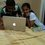# What do you think 2/0 should be?

If I ask you to give the answer for 2/0, you'd probably say-INFINITY-right? Wrong. According to your answer, 2/0=infinity Now, that infinity will obviously have a numeric value,so, 2=infinityx0 Anything multiplied by 0 is 0,so, 2=0,which is not possible. Now, you might change your answer to-invalid-right? I have an answer to that too, I say, 2/0=0.2 If we convert it to percentage,then, 2/0x100=20% Then we convert it to numerical value, which is, 0.2 Do you agree? Your comments an suggestions are welcomed.Note by Amisha Gupta
7 years, 2 months ago

This discussion board is a place to discuss our Daily Challenges and the math and science related to those challenges. Explanations are more than just a solution — they should explain the steps and thinking strategies that you used to obtain the solution. Comments should further the discussion of math and science.

When posting on Brilliant:

• Use the emojis to react to an explanation, whether you're congratulating a job well done , or just really confused .
• Ask specific questions about the challenge or the steps in somebody's explanation. Well-posed questions can add a lot to the discussion, but posting "I don't understand!" doesn't help anyone.
• Try to contribute something new to the discussion, whether it is an extension, generalization or other idea related to the challenge.

MarkdownAppears as
*italics* or _italics_ italics
**bold** or __bold__ bold
- bulleted- list
• bulleted
• list
1. numbered2. list
1. numbered
2. list
Note: you must add a full line of space before and after lists for them to show up correctly
paragraph 1paragraph 2

paragraph 1

paragraph 2

[example link](https://brilliant.org)example link
> This is a quote
This is a quote
    # I indented these lines
# 4 spaces, and now they show
# up as a code block.

print "hello world"
# I indented these lines
# 4 spaces, and now they show
# up as a code block.

print "hello world"
MathAppears as
Remember to wrap math in $$ ... $$ or $ ... $ to ensure proper formatting.
2 \times 3 $2 \times 3$
2^{34} $2^{34}$
a_{i-1} $a_{i-1}$
\frac{2}{3} $\frac{2}{3}$
\sqrt{2} $\sqrt{2}$
\sum_{i=1}^3 $\sum_{i=1}^3$
\sin \theta $\sin \theta$
\boxed{123} $\boxed{123}$

Sort by:

Actually, your second line has some glitch.You are saying ∞ will have some a numeric value.∞ is not a number.It is a denotation that tells that when you will keep going you'll never reach your destination, literally. 2 divided by 0 is undefined.You can't do any mathematical operation ahead of this. Read this wiki : https://en.wikipedia.org/wiki/Infinity

- 3 years, 11 months ago

I would say 2/0 is not defined . And also .. infinity is not any number actually so it will never have a numeric value .

- 7 years ago

Dear friends, the question is do you agree with the above equation.

- 7 years, 2 months ago

We never say 2/0= infinity. We always say 2/0= not defined. Since the value cannot be defined

- 7 years, 2 months ago

Thank you for your statement. 2/0=infinity is for those who think its correct to say like that. I am sure you are not one of them.

- 7 years, 2 months ago

Why have u taken 2/0=.2??

- 7 years, 2 months ago

Thank you for your question. 2/0=0.2 is what I am prooving.

- 7 years, 2 months ago

Now I understand your point. You cancelled 0 by the 0 of 100!!! I was not able to get how u did that statement. Thats wrong u cannot do that.

- 7 years, 2 months ago

Can you tell me why?

- 7 years, 2 months ago

The cancellation of zero means both the numbers have 10 as a common factor, so we divide both numbers by 10 by cancelling the zeroes. Division by zero is not defined.

- 7 years, 2 months ago

Look, its very simple you cannot a common factor of a number which is neither considered a natural number or a whole number,

- 7 years, 2 months ago

0.infinity is undeterminte form so u can't say like 0.infinity =0.

- 7 years, 2 months ago

Thank you for comment. I am not saying that infinity=0. I am saying, infinity,to an extent,will obviously have a value. That value is thereby is being taken here.

- 7 years, 2 months ago